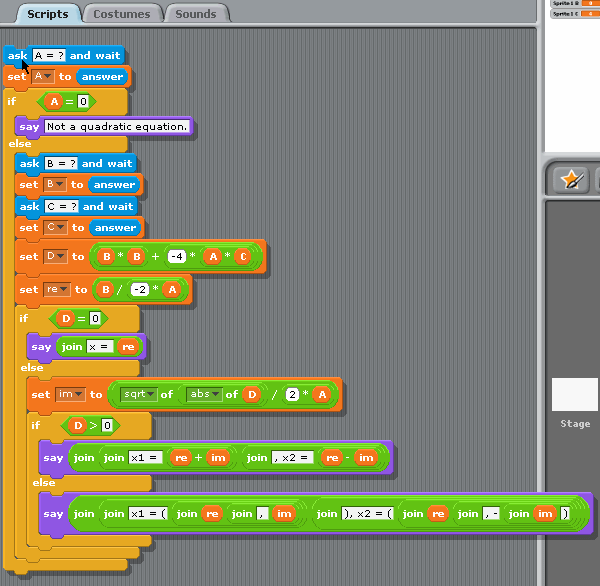Example for versions Scratch 1.4

Scratch is a graphical language, the print-screen contains the actual information about the program, source text is only a transcription. This example features the usage of text input in Scratch, which is done with command `ask "..." and wait`. This command makes the sprite “say” the associated message (used as prompt) and displays an input window below the sprite. Once the information is entered, is can be accessed through predefined variable `answer`, which is copied to local variables before entering next values.

``````ask "A = ?" and wait
if A = 0
else
ask "B = ?" and wait
ask "C = ?" and wait
set D to B*B + (-4)*A*C
set re to B/(-2*A)
if D = 0
say join ("x = " re)
else
set im to (sqrt of (abs of D)) / (2*A)
if D > 0
say join (join ("x1 = " (re+im)) join (", x2 = " (re-im)))
else
say join (join ("x1 = (" join (re join (", " im))) join ("), x2 = (" join (re join (", -" join (im ")")))))
``````